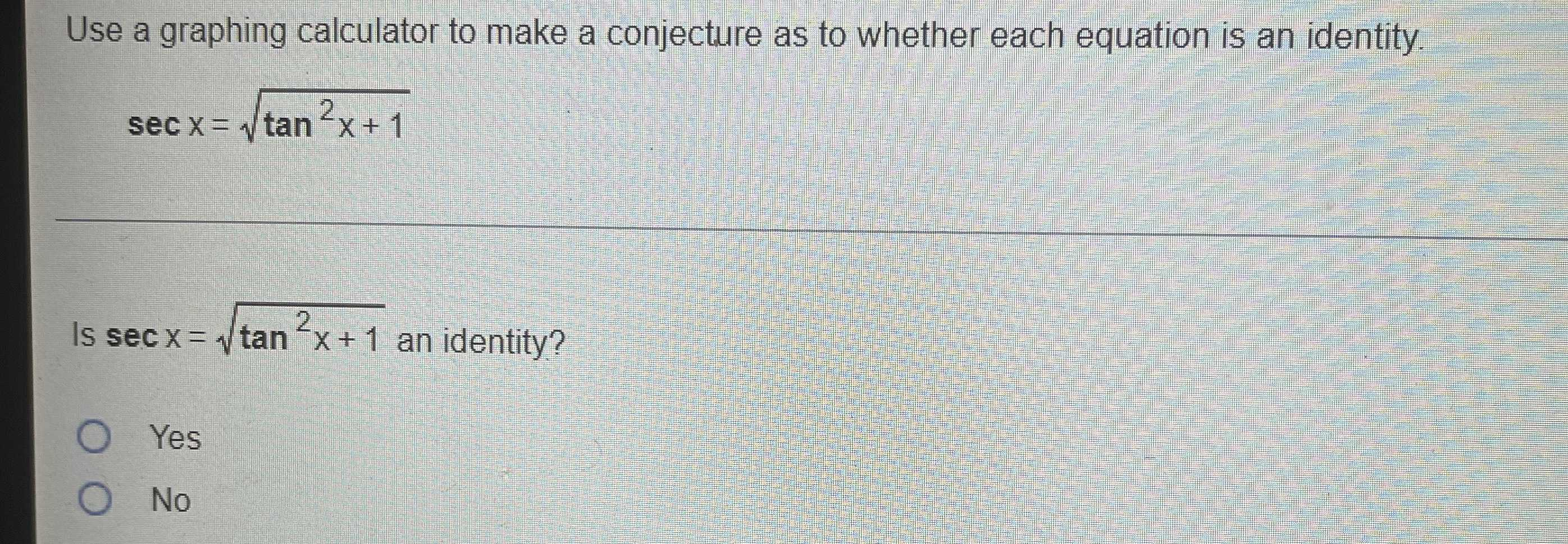### Still have math questions?

Trigonometry
QuestionUse a graphing calculator to make a conjecture as to whether each equation is an identity.

$$\sec x = \sqrt { \tan ^ { 2 } x + 1 }$$

Is $$\sec x = \sqrt { \tan ^ { 2 } x + 1 }$$ an identity?

Yes

No